# Complexity Analysis & Big O

O(1) < O(logn) < O(n) < O(nlogn) < O(n^2) < O(2^n) < O(n!)

# if the value of n is large, (which it usually is, when we are considering Big O ie worst case), logn can be greater than 1


The following are examples of common complexities and their Big O notations, ordered from fastest to slowest:

• Constant: O(1)
• Logarithmic: O(log(n))
• Linear: O(n)
• Log-linear: O(nlog(n))
• Cubic: O(n^3)
• Exponential: O(2^n)
• Factorial: O(n!)

Note that in the context of coding interviews, Big O notation is usually understood to describe the worst-case complexity of an algorithm, even though the worst-case complexity might differ from the average-case complexity.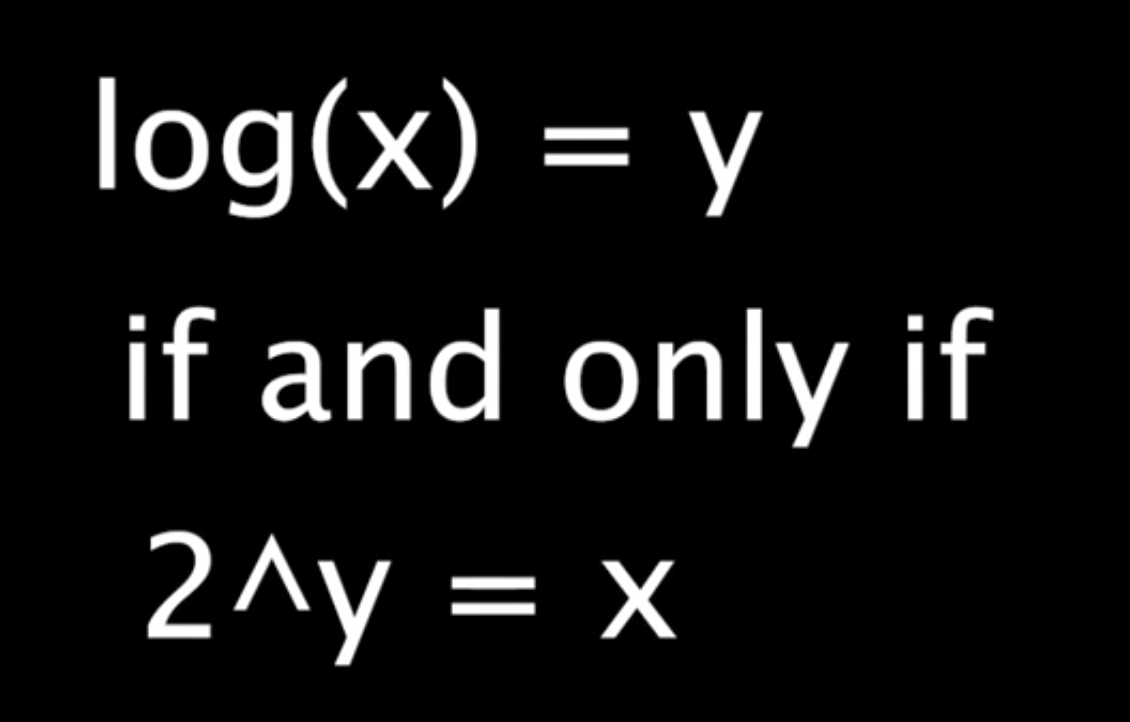## Important rules to know with Big O

### 1. Different steps get added

# O(a+b)
def do_something():
do_step_one() # O(a)
do_step_two() # O(b)


### 2. Drop constants

# O(2n) => O(n)


### 3. Different inputs ⇒ Different variables

# O(a.b)
def random(array_a, array_b):
...


### 4. Drop non-dominant terms

# O(n^2)
def do_something(array):
do_step_one(array) # O(n)
do_step_two(array) # O(n^2)


### 5. Multi-Part Algorithms: Add vs. Multiply

If your algorithm is in the form "do this, then, when you're all done, do that" then you add the runtimes.

If your algorithm is in the form "do this for each time you do that" then you multiply the runtimes.

### 6. Recursive Runtimes

Try to remember this pattern. When you have a recursive function that makes multiple calls, the runtime will often (but not always) look like O( branches ^ depth ), where branches is the number of times each recursive call branches.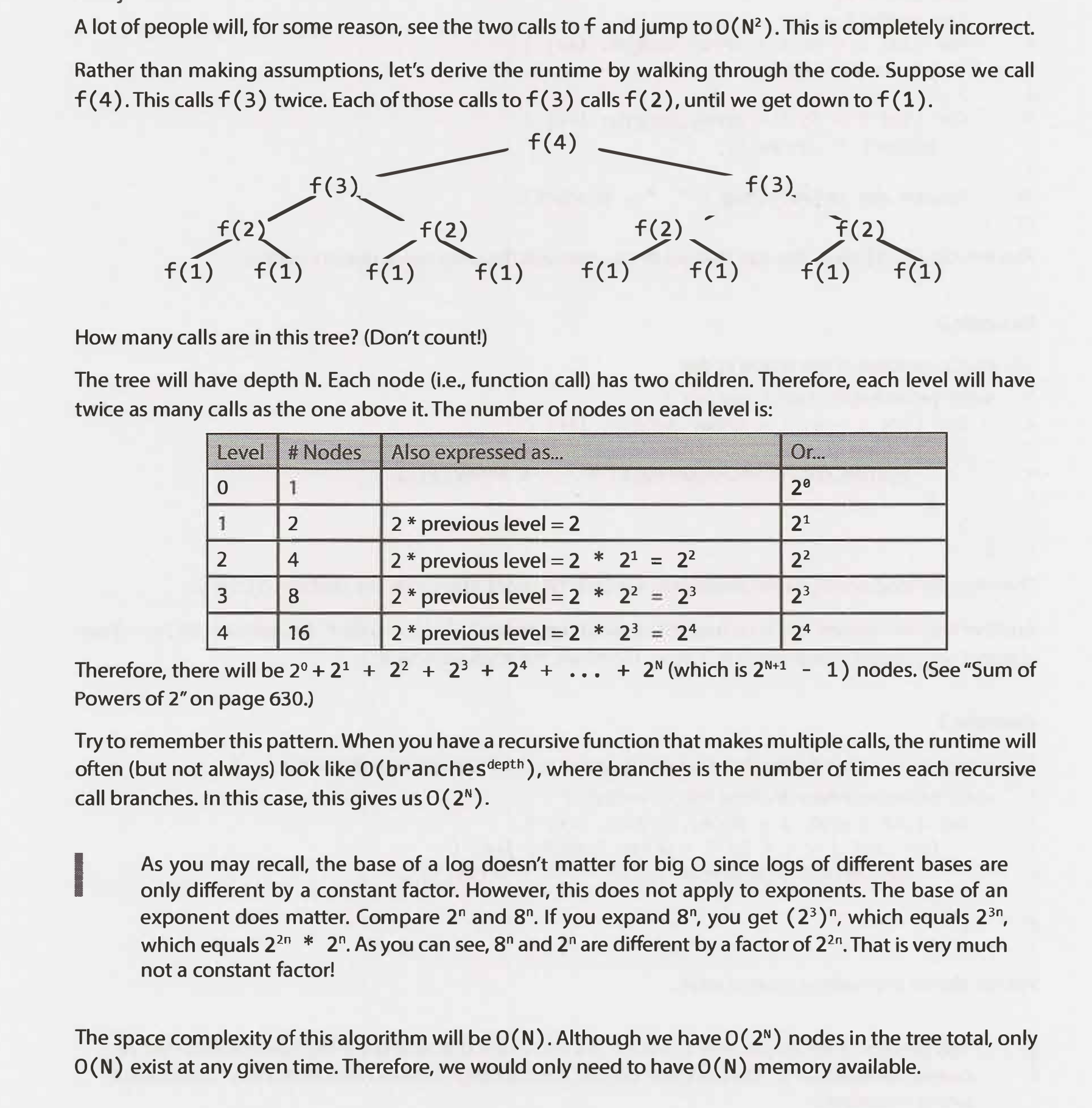## Memory

Accessing a memory slot is a very basic operation that takes constant time. For example, Accessing a value in an array at a given index → array

## Constant time/space complexity:

No matter how you change the input size, the time it takes to get your output is the same. With constant space complexity, the amount of memory you use doesn’t change as the input size grows. Examples:

• Basic Math Operators (+, -, *, /, %)
• Array Index Lookups (arr)
• Hash Map Get/Set Operations (map.get(“key”), map.set(“key”, “value”))
• Property Lookups (array.length, list.head, node.value…)
• Class Instantiations (let c = new Circle(radius = 5, colour = “blue”))

There are also a number of operations that typically fall in this category if implemented most efficiently. Usually, they boil down to doing one or more of the previously mentioned operations, like instantiating an object or looking up some property.

Some examples include:

• Stack push(), pop(), peek()
• Queue enqueue(), dequeue()
• Binary Tree Node getleftChild(), getrightChild()
• Graph Node getAdjacentNodes(node), connectNodes(node1, node2)

A lot of engineers get tripped up by O(1) space, but all this means the amount of memory you use doesn’t scale with the input size.

### A Wrinkle (finite character set)

Example:

Suppose you wanted to write a function that counted all the frequencies of characters in a string. A really simple (and efficient) way to do it would be to loop through all of the string’s characters and then tally them up in a hash map:

The runtime of this function depends on the length of the string. 1 more character, means 1 more step, so the runtime is O(N).

But what about space complexity? We’re using a few variables, but the obvious one that seems to scale up with the size of the string is the counts hashmap. The more characters you have, the more it seems like you’ll have to keep track of, so it might seem like it’s also O(N).

But the reality is that it’s actually O(1) 🤯 because you are only dealing with a finite character set. Sometimes see this big-O notation written as O(C). It’s used to express the fact that constant time/space isn’t just limited to one step or one unit of memory usage. There can be some amount of scaling up in runtime or memory usage, as long as it’s to some fixed, finite upper limit.

## Examples

### Logarithm

A mathematical concept that's widely used in Computer Science and that's defined by the following equation:

In the context of coding interviews, the logarithm is used to describe the complexity analysis of algorithms, and its usage always implies a logarithm of base 2. In other words, the logarithm used in the context of coding interviews is defined by the following equation:

In plain English, if an algorithm has a logarithmic time complexity O(log(n), where n is the size of the input), then whenever the algorithm's input doubles in size (i.e., whenever n doubles), the number of operations needed to complete the algorithm only increases by one unit. Conversely, an algorithm with a linear time complexity would see its number of operations double if its input size doubled.

As an example, a linear-time-complexity algorithm with an input of size 1,000 might take roughly 1,000 operations to complete, whereas a logarithmic-time-complexity algorithm with the same input would take roughly 10 operations to complete, since 210 ~= 1,000.

### 2n (2^n) - whenever the algorithm's input increases in size, the number of operations needed to complete the algorithm doubles**.

If n is the size of the input), then whenever the algorithm's input increases in size (i.e., n+1), the number of operations needed to complete the algorithm doubles.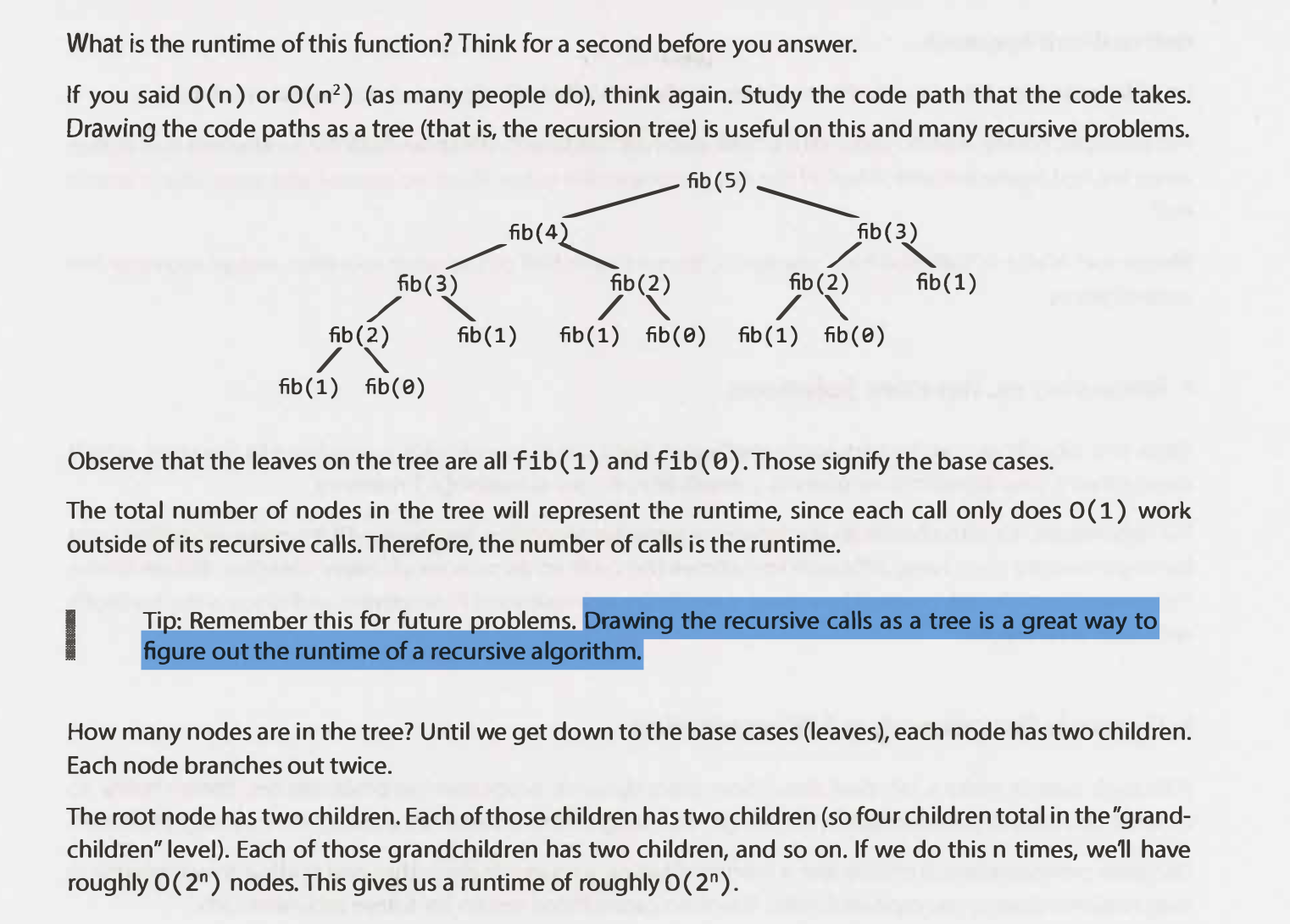### Factorial - N * (N-1) * (N-2) * (N-3) * ... * 2 * 1 == N!

Factorial - Wikipedia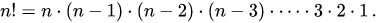The factorial function (symbol: !) says to multiply all whole numbers from our chosen number down to 1.

Examples:

4! = 4 × 3 × 2 × 1 = 24

7! = 7 × 6 × 5 × 4 × 3 × 2 × 1 = 5040

1! = 1

### Not factorial 😂 - (N-1) + (N-2) + (N-3) + ... + 2 + 1 ~= N^2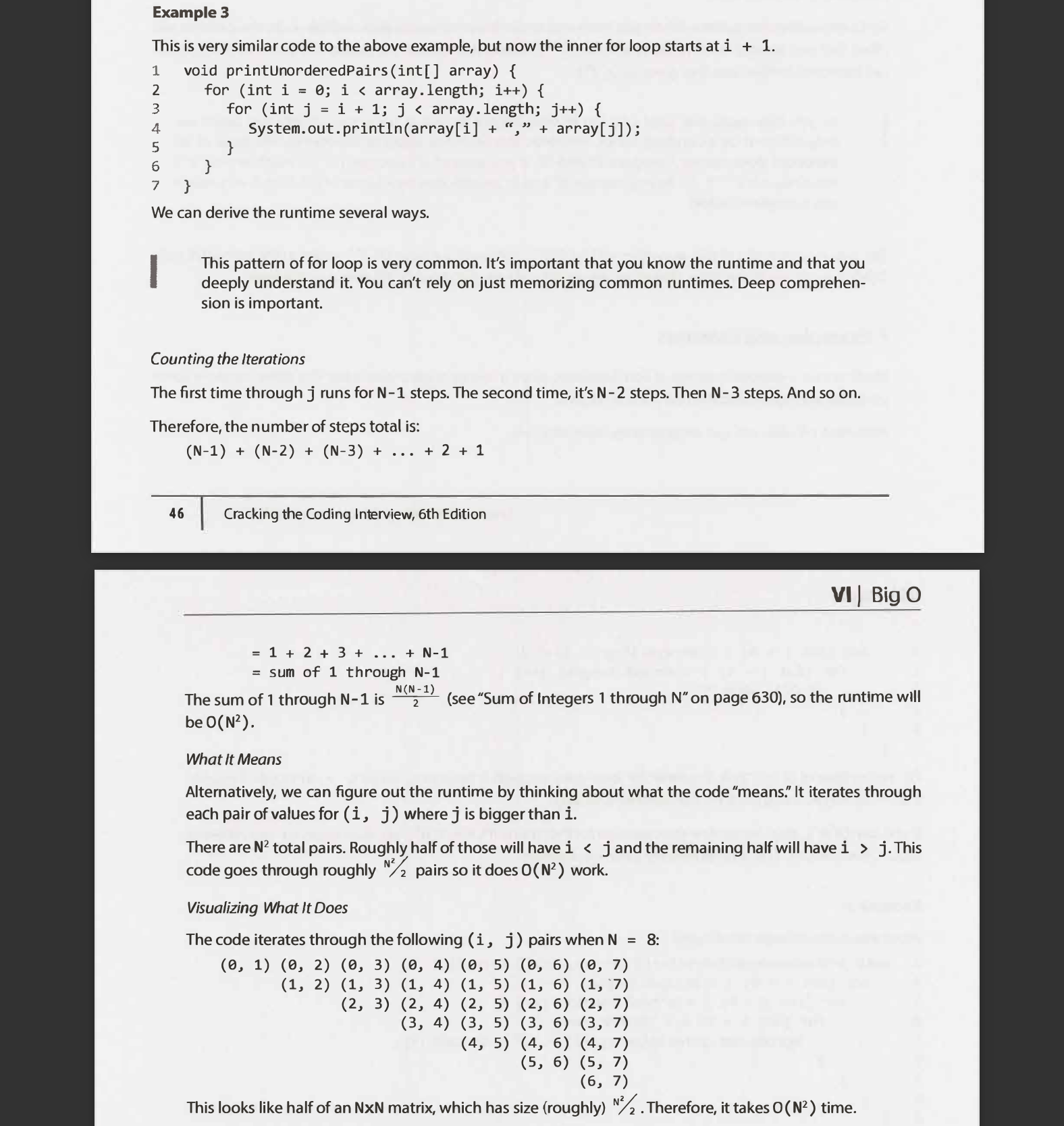### Two loops but on different arrays O(a.b) instead of O(n^2)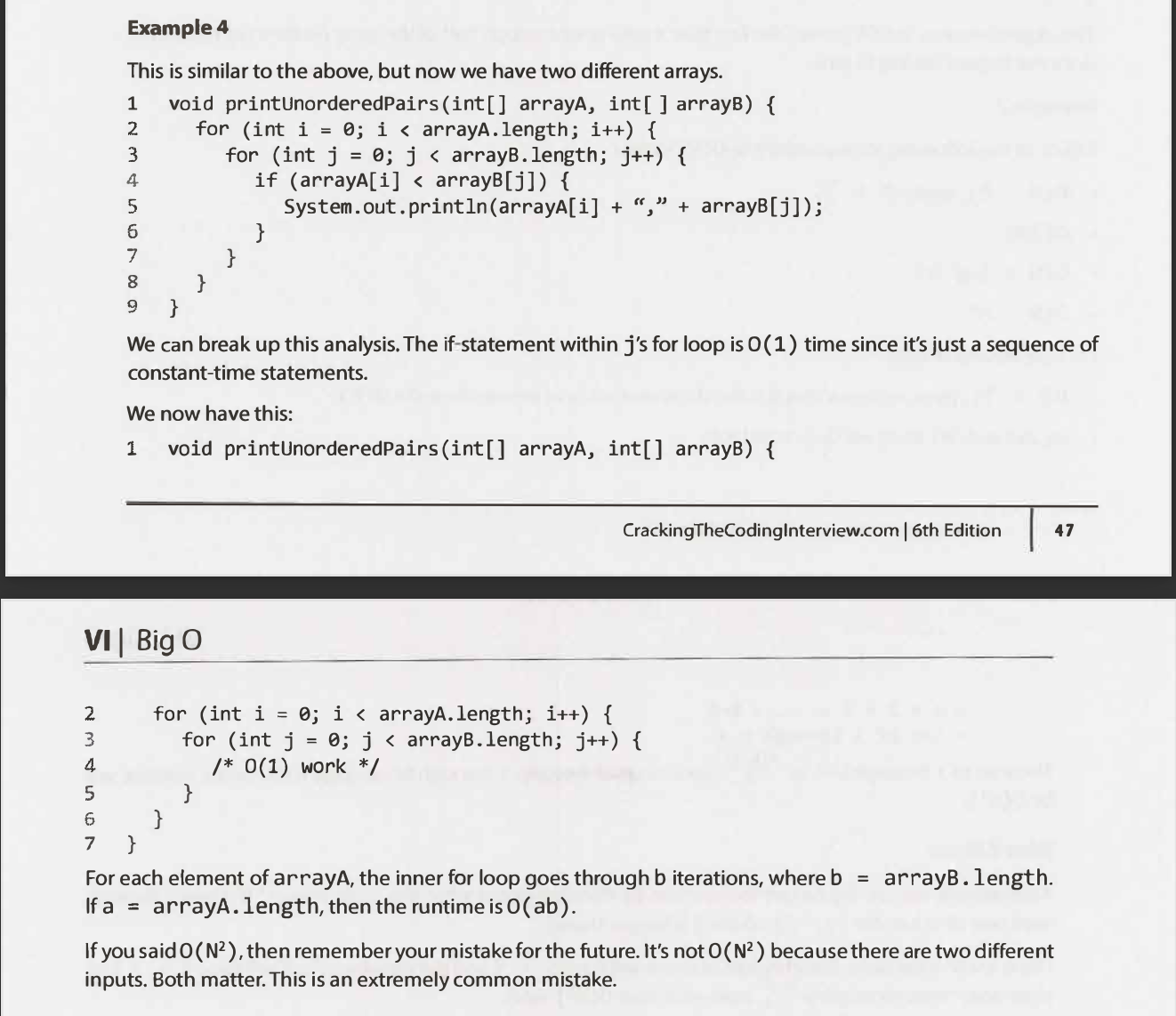### An algorithm that took in an array of strings, sorted each string and then sorted the full array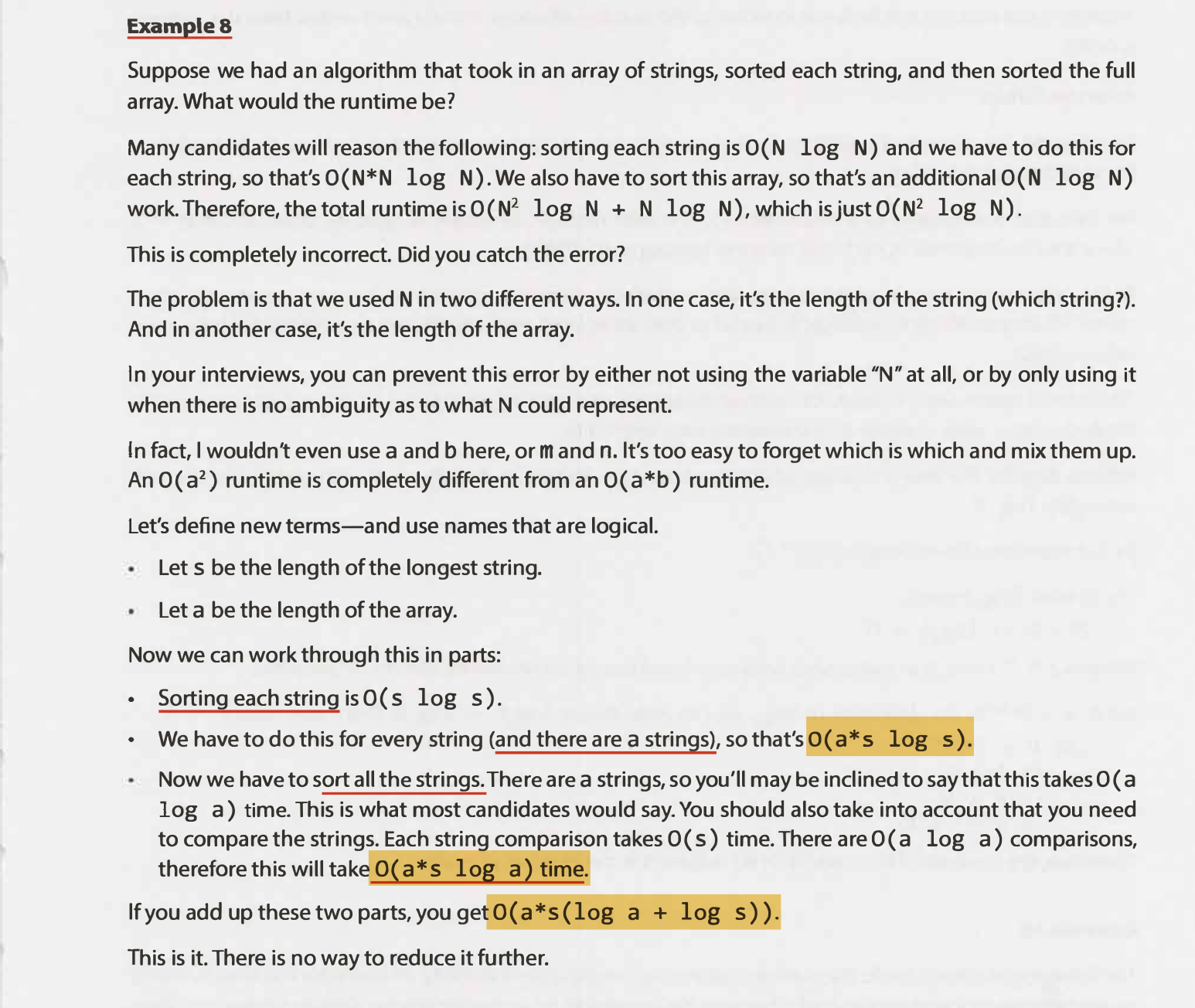### O(squareroot n) time.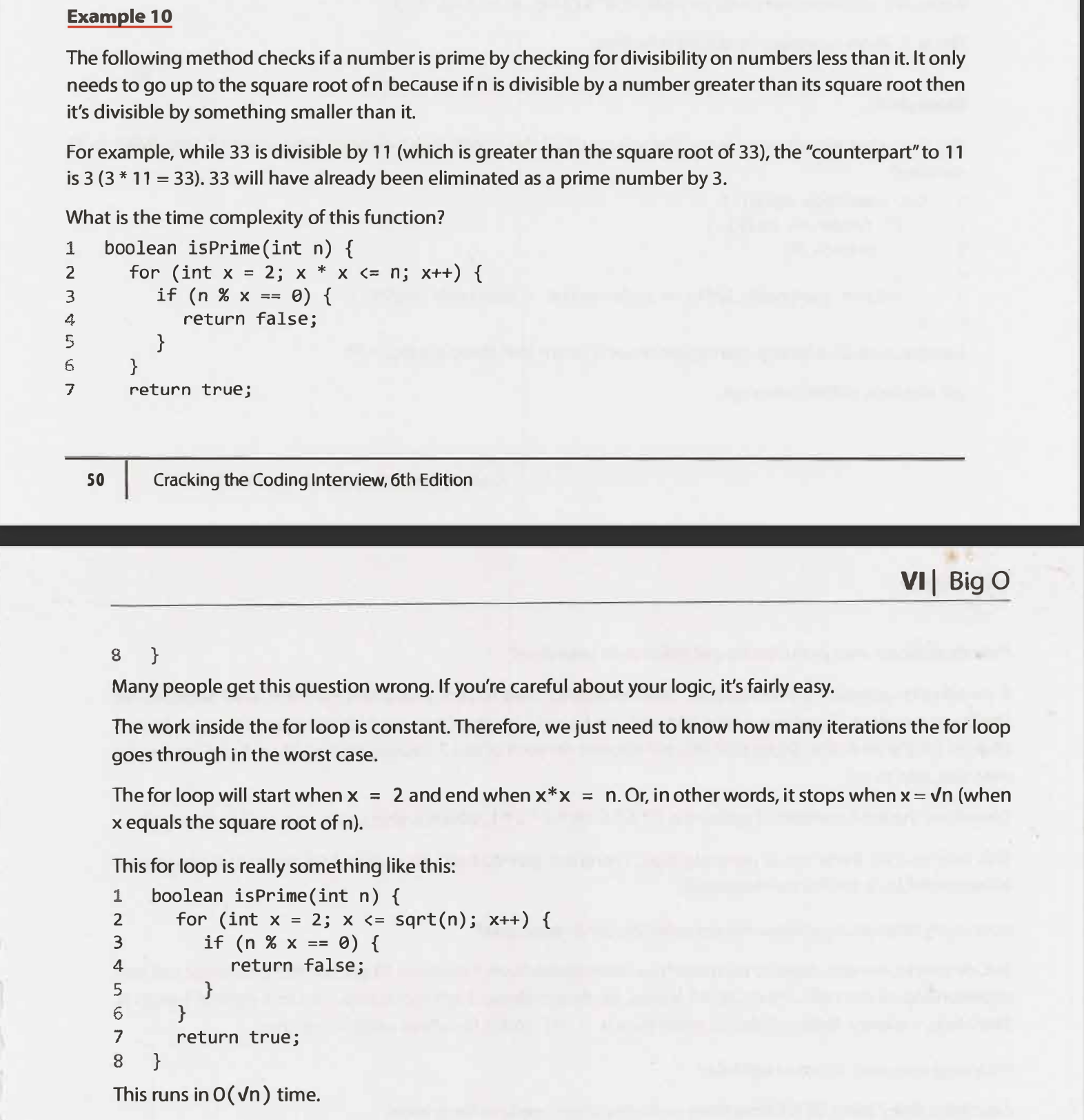## Common

### Graph DFS & BFS

Depth First Search or DFS for a Graph - GeeksforGeeks

The time complexity of DFS & BFS if the entire tree is traversed is O(V) where V is the number of nodes. In the case of a graph, the time complexity is where V is the number of vertexes and E is the number of edges.

The Time complexity of BFS is O(V+E) when Adjacency List is used and O(V^2) when Adjacency Matrix is used, where V stands for vertices and E stands for edges.